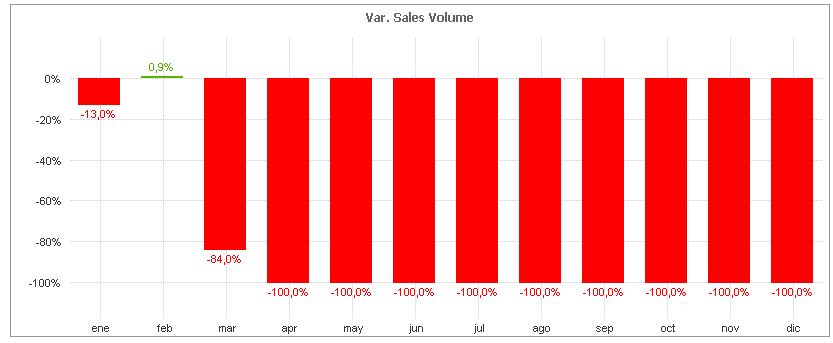# QlikView App Dev

Discussion Board for collaboration related to QlikView App Development.

Announcements
Become an analytics expert with Qlik's new 15 week course: Applied Data Analytics using Qlik Sense. READ MORE
cancel
Showing results for
Did you mean:Creator II

## Bar Chart Columns Visibility

Hello guys,

Currently I'm working in a new dashboard and I'm kind of blocked with a Bar Char property:

I have this expression in this chart:

(Sum({<Año = {\$(vMaxYear)}>} Hl) / Sum({<Año = {\$(=\$(vMaxYear)-1)}>} Hl))-1And I don't want to show the bars that are showing -100% due to the lack of data; for april 2018 I don't have any so the division is zero so I want to show it as a zero bar (or if there is another solution).

How can I do this?

Thank you very very much.

1 Solution

Accepted SolutionsMVP

May be this

If(Sum({<Año = {\$(vMaxYear)}>} Hl) <> 0,

(Sum({<Año = {\$(vMaxYear)}>} Hl) / Sum({<Año = {\$(=\$(vMaxYear)-1)}>} Hl))-1)

2 RepliesMVP

May be this

If(Sum({<Año = {\$(vMaxYear)}>} Hl) <> 0,

(Sum({<Año = {\$(vMaxYear)}>} Hl) / Sum({<Año = {\$(=\$(vMaxYear)-1)}>} Hl))-1)Creator II
Author

Awesome!!! worked perfect.Then in presentation I unchecked "suppress Zero-values" and checked Zero on bars so I can have this: